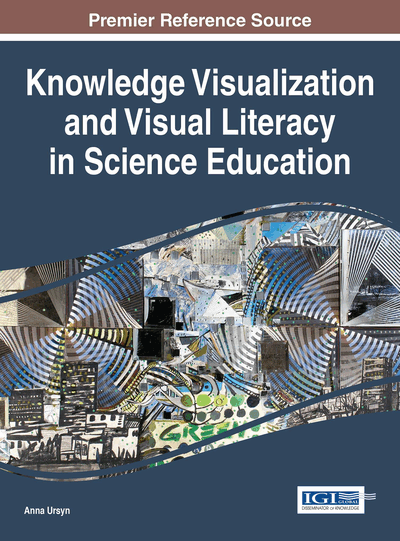# Visualisation and Communication in Mathematics

Hervé Lehning (AC-HL, France)
Copyright: © 2016 |Pages: 19
DOI: 10.4018/978-1-5225-0480-1.ch006
OnDemand PDF Download:
Available
\$37.50
No Current Special Offers

## Abstract

Communication in mathematics is necessary at several levels: popularisation, teaching and research. Even a small drawing is useful in every case: to set a problem, to understand it, to find a method to tackle it and to illustrate it. An appealing aesthetic can also draw the attention on the subject.
Chapter Preview
Top

## Missing Points

In this first example, the visualisation is illustrating the proof rather than giving creative ideas. In similar cases, ideas come when we consider points a priori missing in the problem, but giving new lights on it. For example, to show that the three altitudes of a triangle are concurrent, we just need to construct another triangle in which these lines are the perpendicular bisectors (Figure 1).

A subtler problem, posed by Pierre de Fermat in 1636, changes when introducing new points:

In a given triangle ABC, locate a point whose distances from A, B and C have the smallest possible sum.

A simple idea is to bring back the question to the following result: “the shortest way between two points is the straight line”. Thus, given a point M, we look for a point D such that the sum AM + BM + CM is equal to the length of a broken line connecting C and D. The idea comes when constructing an equilateral triangle ABD on side AB. This idea leads to some extra conditions (all angles of the triangle are less than 120° and B is the point where the angle is maximum) but remains rather general.

To answer the question, point M must be located on lines CD and AE (where E is the point similar to D construct on BC) thus at their intersection. The proof follows: if P is a point of the triangle ABC, the sum AP + BP + CP is equal to the length of the broken line CPQD, which is minimal if P is on line CD, and the same on AE (Figure 2).

Top

## Sangaku

If visualising a problem is particularly helpful in geometry to determine where this problem stands and to discover ideas leading to a solution, it also allows setting it in an enigmatic and aesthetic way. Let us see the difference between the approaches on a small exercise at high school level, written in a classical form:

Two circles externally tangent and a straight line tangent to both of them are given. Show that: AB2 = 4 rR where A and B are the points of tangency on the line, R and r the radiuses of the two circles.

Visually presented, the exercise is immediately intelligible without preliminary work and far more exciting too. Thus, everyone has a direct access to the question, which becomes a kind of enigma, exciting to tackle. It leads us to the Japanese Sangaku, which are little masterpieces at the level of mathematical reasoning as well as the level of visual aesthetics (Fukagawa & Rothman, 2008; Huvent, 2008). The key idea to solve it can be given through a small drawing, introducing the right-angled triangle CDE, which leads to use Pythagoras’ theorem. This gives directly: (R + r)2 = (R – r)2 + AB2 and then the result (Figure 3).

Here, we meet the spirit of the Japanese Sangakus, which are small masterpieces at both mathematical and aesthetic level. Jean Constant (2014) for example, made it an artistic speciality (Figure 4).

## Complete Chapter List

Search this Book:
Reset